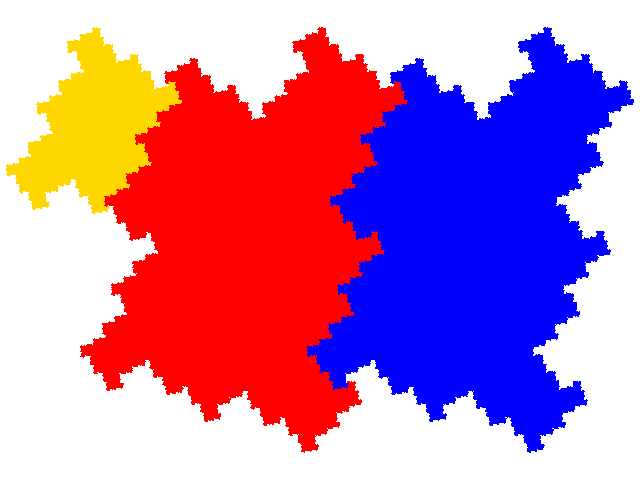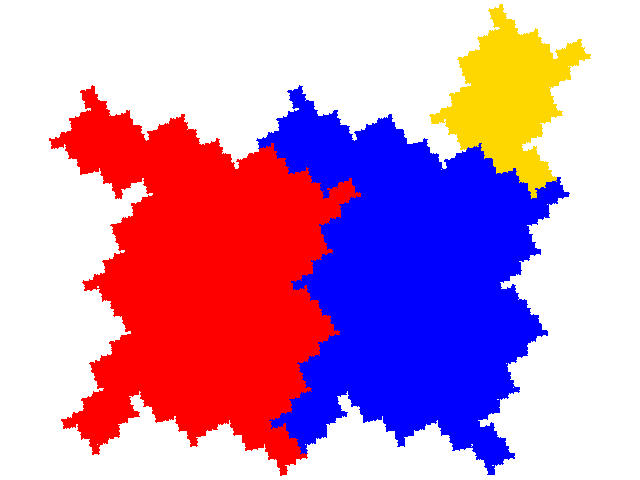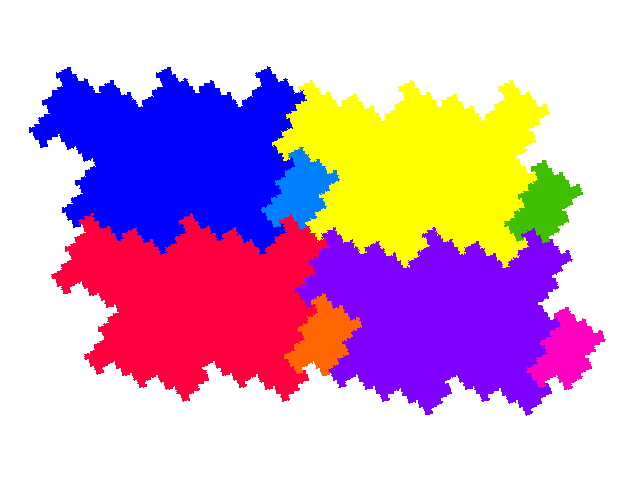# Some tiles associated with the 6th unit cubic Pisot number

## External Tiles

Of the eight order 3 6th cubic tiles, two (the symmetric and windowed tiles) have the smaller element lying within the larger two elements, two (the complex teragons) have it lying partially within and partially outside, and four have it lying outside. Two of these are demi-symmetric tiles, which makes it convenient to refer to the remaining two as external tiles. The IFS for the first attractor shown below is { ```p→-ap; p→-ap + 1; p→-a3p + 2a2``` }. Another IFS, with an attractor with a different orientation, is { ```p→-a3p; p→-ap + 1; p→-ap + 1 + a2``` }. The IFS for the second attractor is { ```p→ap; p→ap + 1; p→a3p + 1 - a-1 - a2``` }.#### Tiling

For the first tile there is a tiling with one copy in the unit cell (signature 0). There are also tilings with other signatures (e.g. 03, 033, 0333). For the tiling with signature 0 the tiling vectors are `2 + a` and `a-1`. For the tiling with signature 03 the cell transforms are { ```p→p; p→a3p + 1 - a1 - a2 - a3``` } and the tiling vectors are ```2 + 2a + a-1``` and `a-1`. For the tiling with signature 033 the cell transforms are { ```p→p; p→a3p + 1 - a1 - a2 - a3; p→a3p + 1 - a2 - a3``` } and the tiling vectors are ```2 + 3a + 2a-1``` and `a-1`. For the tiling with signature 0333 the cell transforms are { ```p→p; p→a3p + 1 - a1 - a2 - a3; p→a3p + 1 - a2 - a3; p→a3p + 1 - 2a2 - a3``` } and the tiling vectors are ```2 + 4a + 2a-1``` and `a-1`. Obviously this series can be extended for all signatures 03n. Other simple tilings have signatures 002 (dissecting each unit cell of 0), 0113 (dissecting alternative unit cells along rows, or columns, or diagonals), 0022 (dissecting the larger copy in 03) and 0446 (dissecting the smaller copy in 03).For the second tile there is again a tiling with signature 0. The tiling vectors are `2 + a` and `a-1`. There are no obvious "pull apart" tilings, but tilings with signatures like 002 and 0113 can be obtained by dissection.© 2015, 2016 Stewart R. Hinsley